# Mechanical work

In physics, mechanical work is the amount of energy transferred by a force. Like energy, it is a scalar quantity, with SI units of joules. Heat conduction is not considered to be a form of work, since there is no macroscopically measurable force, only microscopic forces occurring in atomic collisions. In the 1830s, French mathematician Gaspard-Gustave Coriolis coined the term work for the product of force and distance.

Positive and negative signs of work indicate whether the object exerting the force is transferring energy to some other object, or receiving it. A baseball pitcher, for example, does positive work on the ball, but the catcher does negative work on it. Work can be zero even when there is a force. The centripetal force in uniform circular motion, for example, does zero work because the kinetic energy of the moving object doesn't change. Likewise, when a book sits on a table, the table does no work on the book, because no energy is transferred into or out of the book.

## Calculation

When the force is constant and along the same line as the motion, the work can be calculated by multiplying the force by the distance,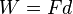$W=Fd$ (letting both F and d have positive or negative signs, according to the coordinate system chosen). When the force does not lie along the same line as the motion, this can be generalized to the scalar product of force and displacement vectors.

In the simplest case, that of a body moving in a steady direction, and acted on by a constant force parallel to that direction, the work is given by these formulas: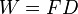$W = F D \,\!$             (1)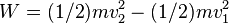$W = (1/2) mv_2 ^2 - (1/2) mv_1 ^2$             (derived simply from the above equation)

where

F is the portion of the force acting in the same direction as the motion, and
D is the distance traveled by the object. Note that distance is a scalar quantity and so, too, is work.
m is the mass of the object
v2 is the final velocity
v1 is the initial velocity

The work is taken to be negative when the force opposes the motion. More generally, the force and distance are taken to be vector quantities, and combined using the dot product: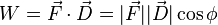$W = \vec{F} \cdot \vec{D} = |\vec{F}| |\vec{D}| \cos\phi$             (2)

where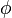$\phi \,$ is the angle between the force and the displacement vector. This formula holds true even when the object changes its direction of travel throughout the motion.

In situations in which the force changes over time, and/or the direction of motion changes over time, equation (1) is not directly applicable. However, under mild restrictions, it is possible to divide the motion into small steps, such that the force and motion are well approximated as being constant for each step, and then to express the overall work as the sum over these steps. This is formalized by the following line integral, which can be taken as a rather general definition of work: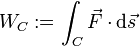$W_C := \int_{C} \vec{F} \cdot \mathrm{d}\vec{s}$             (3)

where:

C is the path or curve traversed by the object$\vec F$ is the force vector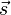$\vec s$ is the position vector

It must be emphasized that$W_C\,$ is explicitly a function of the path$C\!$. In general the work$W_C\,$ depends on the total displacement of the path$C\!$ (The longer the path traveled by the object, the more work will be done).

Using vector notation, equation (3) readily explains how a nonzero force can do zero work. The simplest case is where the force is always perpendicular to the direction of motion, making the integrand always zero (as is the case in circular motion).

The possibility of a nonzero force doing zero work exemplifies the difference between work and a related quantity, impulse, which is the integral of force over time. Impulse measures change in a body's momentum, a vector quantity sensitive to direction, whereas work considers only the magnitude of the velocity. For instance, as an object in uniform circular motion traverses half of a revolution, its centripetal force does no work, but it transfers a nonzero impulse.

## Units

The SI unit of work is the joule (J), which is defined as the work done by a force of one newton acting over a distance of one meter. This definition is based on Sadi Carnot's 1824 definition of work as "weight lifted through a height," which is based on the fact that early steam engines were principally used to lift buckets of water, though a gravitational height, out of flooded ore mines. The dimensionally equivalent newton-meter (N•m) is sometimes used instead; however, it is also sometimes reserved for torque to distinguish its units from work or energy.

Non-SI units of work include the erg, the foot-pound, the foot-poundal, and the liter-atmosphere.

## Types of work

Forms of work that are not evidently mechanical in fact represent special cases of this principle. For instance, in the case of "electrical work," an electric field does work on charged particles as they move through a medium.

One mechanism of heat conduction is collisions between fast-moving atoms in a warm body with slow-moving atoms in a cold body. Although colliding atoms do work on each other, the force averages to nearly zero in bulk, so conduction is not considered to be mechanical work.

### Work due to volume change

Work is done when the volume of a fluid changes. Work in such circumstances is represented by the following equation:$W_C =-\int_C P\,\mathrm{d}V$           (4)

where:

• W = work done on the system
• P = external pressure
• V = volume

Like all work functions, PV work is dependent on the path$C\!$. PV work is often measured in the (non-SI) units of liter-atmospheres, where 1 L•atm = 101.3 J.

## Mechanical energy

The mechanical energy of a body is that part of its total energy which is subject to change by mechanical work. It includes kinetic energy and potential energy. Some notable forms of energy that it does not include are thermal energy (which can be increased by frictional work, but not easily decreased) and rest energy (which is constant as long as the rest mass remains the same).

### The relation between work and kinetic energy

If an external work W acts upon a body, causing its kinetic energy to change from Ek1 to Ek2, then: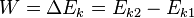$W = \Delta E_k = E_{k2} - E_{k1}\,\!$

Also, if we substitute the equation for kinetic energy that states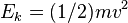$E_k = (1/2) mv^2$, we then get: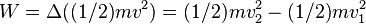$W = \Delta ((1/2) mv^2) = (1/2) mv_2 ^2 - (1/2) mv_1 ^2$

### Conservation of mechanical energy

The principle of conservation of mechanical energy states that, if a system is subject only to conservative forces (such as a gravitational force), its total mechanical energy remains constant.

For instance, if an object with constant mass is in free fall, the total energy of position 1 will equal that of position 2.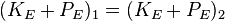$(K_E + P_E)_1 = (K_E + P_E)_2 \,\!$

where

•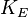$K_E$ is the kinetic energy, and
•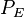$P_E$ is the potential energy.

The external work will usually be done by the friction force between the system on the motion or the internal, non-conservative force in the system, or loss of energy due to heat.